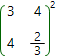# Matrix power online calculatorTo find matrix A raised in n - th power, where n is natural number, one should multiply matrix A by itself n times. In addition,

A0 = E,

where E - identity matrix.

Power operation can only be applied to the square matrix. This conclusion immediately follows from the matrices multiplication rule.

Matrix power calculatorA =